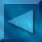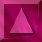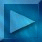Previous : What is the problem?  Next : Purpose of the regularization Notations

 Reconstruction - Regularization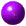Least squares
A simple solution for image restoration = optimizing the least squares criterion.
Calculate the image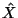which minimizes the function J(X) :

J(X)=|| Y-HX || 2

This inverse problem is ill-posed (in the sense of Hadamard) :

• the solution is not unique ;
• it may be unstable.
• Reconstruction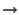noise amplification

Small variations of  the observed image Yhigh variations of the reconstructed solution X.

Example (synthetic image) :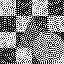Original image Corrupted image Reconstructed solutionRegularization

 Calculate the imagewhich minimizes the energy U(X) :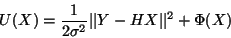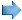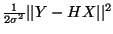=  data-dependent term (Y=data)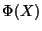=  regularization term, which penalizes noisy solutions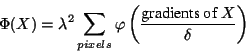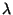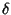= hyperparameters of the model

gradients of X = differences between neighbour pixels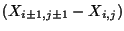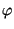= Phi-function, it takes into account the constraints imposed on the solution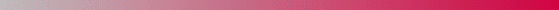André Jalobeanu - 24 Aug 1998